Gábor Erdos

## Substitutions and Replacements in Mechanism PrototypingNBCDFPDF

In parameterized mechanism design, there are two contradicting requirements: keep the governing equation as general as possible (everything in symbolic form), but be able to quickly look at (or simulate) the mechanism and see how it moves at every stage of the design process (which requires numeric representation). Handling symbolic and numerical representations together is a basic paradigm of symbolic manipulator programs like Mathematica. The solutions developed for this paradigm can be used for enhancing the flexibility of a mechanism prototyping application package written in Mathematica. This article shows some examples of mechanism modeling with the LinkageDesigner application package that uses this bundled symbolic and numeric representation. The bridge between the two representations is substitution, enabling a one-way route from symbolic to numeric representation. A historical parabola-drawing mechanism and the spirograph will be used as examples. Besides the power of substitution, replacement is also widely used in LinkageDesigner. This simple tool can be handy in solving problems like the inverse kinematic problem (IKP). Two examples will be considered: the IKP of a 6R robot and a parallel mechanism with three degrees of freedom.

### Introduction

Parameterized mechanism modeling appeals to designers because it can greatly simplify the design process. Mechanism design is an iterative process; since the specifications are continually changing, the mechanism should adopt them. Parametrized mechanism models can support the evolution of a design if changes in the specification can be achieved with small adaptations of the parameters. The theory of computer-aided mechanism modeling distinguishes two main techniques: augmented and embedded. The first approach models the configuration of the mechanism by a vector of Cartesian coordinates that describes the locations and orientations of the links relative to the reference frame. In the embedded approach, generalized—or joint—coordinates are used to specify the postures of the links. Both modeling techniques can handle parameterized mechanisms to some extent; however, the selected modeling technique naturally influences the data model of the mechanism, the type of solver employed, and also the type of problems that can be effectively addressed.

In the augmented technique, the topology of the mechanism is flattened because the position and orientation of every link is maintained relative to the global reference frame. The topology of every mechanism can be modeled with a simple tree, having the ground link in the root and all the moving links connected to the root. Every link of the mechanism is defined with six coordinates (or in the case of a planar mechanism, with three coordinates). The physical constraints of the mechanism (joints) are modeled with a set of constraint equations. The different types of constraints are modeled with a specific number of constraint equations (e.g., a hinge imposes five constraint equations) . Redundant constraint equations are created if the mechanism contains loops. For example, a loop-closing rotational joint might impose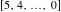independent constraint equations depending on the actual configuration of the mechanism. The topology of the links is not taken into consideration because the constraint equations are usually not all independent. This implies that the mechanism cannot be solved with an ordinary root-finding algorithm like FindRoot, so it requires a special solver. This special solver is inherently numerical ; therefore, a parametrized mechanism—where the constraint equations are generated with the parameters—should be converted to numerical terms before a posture can be calculated.

The big disadvantage of the augmented method is that it requires a special solver even in the case of kinematic modeling. However, if we could eliminate the redundant constraint equations before solving them, a simple solver like FindRoot could be employed. One natural solution would be to let the user resolve the problem. This way the independent constraint equations can be further processed—even solved in closed form if there is a solution; therefore, they are better suited for parametrized mechanism modeling.

LinkageDesigner uses the embedded notation. In this method the topology matches the kinematic graph of the mechanism. The kinematic graph is an undirected graph where the nodes represent links and the edges the joints between the links. To describe the configuration of a mechanism, the relative transformation between the connected links is attached to the edges of the kinematic graph. The independent variables of these transformations are the driving variables of the mechanism. If the kinematic graph has a loop, the loop-closing kinematic pair is treated differently. Instead of the relative transformation, the set of independent constraint equations is attached to the edges of the graph. Because embedded notation always works with the minimal number of constraint equations and does not require a special solver, it can effectively support the design process even in the case of a complicated multi-looped mechanism.

The parameterized mechanism allows the designer to handle a family of mechanisms together. By substituting the parameters with numerical values, a specific instance of the family is obtained. The following sections present examples modeled with the LinkageDesigner application package, where we can see that building a bridge between the generic and specific representation of a family of mechanisms can be implemented with a simple approach using substitution and replacements.

### Parabola-Drawing Mechanism

Even the ancient Greek mathematicians and engineers invented different kinds of curve-drawing mechanisms to help them solve different design problems. All of these linkages incorporate one special feature of the curves that became the working principle of the mechanism. The following parabola-drawing mechanism, described in , is probably one of the latest inventions in this field. After the appearance of personal computers, these mechanisms were no longer used. The mechanism is based on the following corollary.

Corollary 1. Letbe the line perpendicular to the axisof the parabola (see Figure 1) andbe the distance from the focus to the directrix of the parabola. If the distance offrom the vertex of the parabola is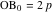, then for an arbitrary point P on the parabola, the angle POB is 90°, where B is the intersection of the linesand, withparallel to.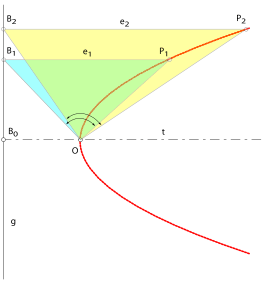Figure 1. Parabola construction.

Corollary 1 implies the working principle of the parabola-drawing mechanism: if a right-angled triangle is rotated in the vertex of the parabola, the third side of the triangle touches the points of the parabola. The mechanism should naturally be designed in such a way that the side BP of the triangle (see Figure 1) should move up and down along lineand its size should be able to shrink or grow. The designed mechanism is shown in Figure 2.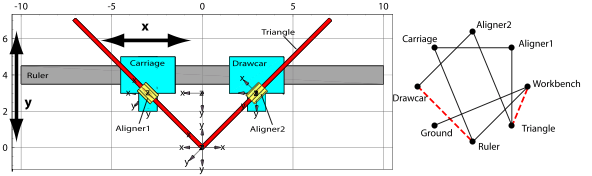Figure 2. Parabola-drawing mechanism.

The mechanism definition is not presented here; only the predefined linkage is used. As mentioned in the Introduction, LinkageDesigner employs the embedded mechanism modeling method. This implies that constraint equations are generated only for loop-closing kinematic pairs. To investigate the equations, load LinkageDesigner and the LinkageData of the parabola-drawing mechanism.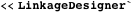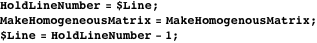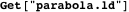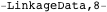The defined mechanism contains two loops, displayed with red dotted lines in Figure 2. The loop-closing constraint equations constrain the joint variables that were independent before. The independent joint variables of the mechanism are stored in the \$DrivingVariables record of the LinkageData, while the implicitly defined (or constrained) joint variables are stored in the \$DerivedParametersB record. Both records store the variable names and their actual substitution values. The \$DerivedParametersB record also stores the constraint equations to be solved.

Here are the generated constraint equations of the mechanism.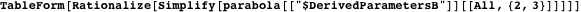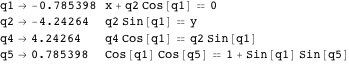These are the driving variables of the mechanism.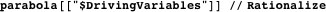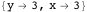The parametric representation of the constraint equation makes it simple to calculate the new posture of the mechanism. If new substitution values of the independent parameters (in this caseand) are assigned, they are substituted into the constraint equations, which become definite and then are solved for the dependent variables. This iterative substitution-solving process is implemented in the AnimateLinkage function to generate an animation of the mechanism if its independent variables are interpolated between the specified limit values.

The mechanism draws a parabola if thedriving variable (i.e., corresponding to the translational position of the Carriage) is set to a fixed value and thedriving variable is interpolated within an interval. Thedriving variable corresponds to the translational position of the Ruler (see Figure 2).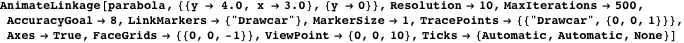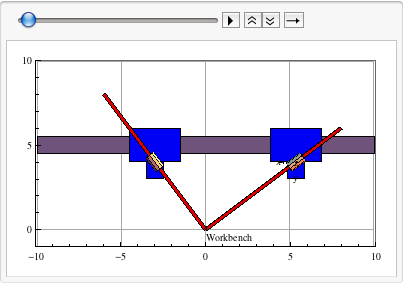### Spirograph

The spirograph is a very simple mechanism consisting of two rigid bodies that are connected by a rolling constraint. The rolling constraint is a higher-order constraint because the general constraint definition requires geometric data of the kinematic pair (rolling curve definitions), unlike the lower-order constraints (e.g., a hinge or translational joint), where a marker’s location fully defines the joint. However, if the rolling curves are circles, the higher-order joint could be easily substituted with two rotational joints because the rolling constraints between circles are very simple.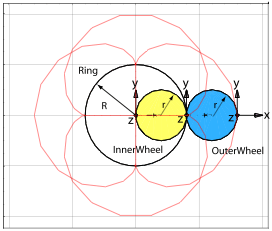Figure 3. Spirograph mechanism.

In this example, a spirograph having an inner and outer planet wheels is built. Both wheels have the same radiusand roll on the same ring of radius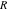. To substitute the rolling constraint, a virtual body is also introduced (called the Arm) that is responsible for rotating the centers of the wheels on circles. This way the rolling constraint is substituted with two rotational joints—the first rotates the Arm around the origin of the Ring, while the second rotates the wheels attached to the arms at distances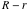and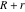s from the origin. The joint variables representing the two rotational joints are not independent because the rolling constraint makes them dependent on each other. This dependency is incorporated into the mechanism during its definition. Because the definition of this mechanism is very short, it is included below.

Create the LinkageData of the mechanism with two geometrical parameters.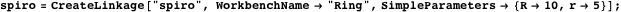Define the rotational joint between Ring and the virtual link Arm.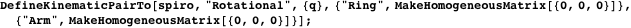Define the rotational joint between Arm and OuterWheel.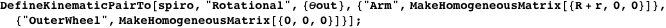Define the rotational joint between Arm and InnerWheel.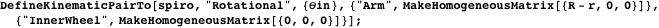So far the mechanism has three degrees of freedom: the three independent joint variables. The rolling constraint can be incorporated in such a way that the rotational joint variablesout andin depend on the radii of the Wheel and the Ring, and the joint variablesof the hinge joint between Ring and Arm. LinkageDesigner provides the function ReplaceDrivingVariables to allow such transactions.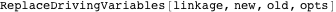moves the old driving variables into \$DerivedParametersA and adds the new driving variables to \$DrivingVariables.

Introduce the rolling constraint of the mechanism.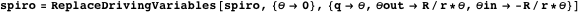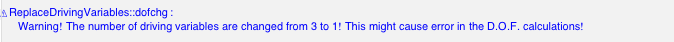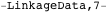Attach geometry to the Ring, InnerWheel, and OuterWheel links.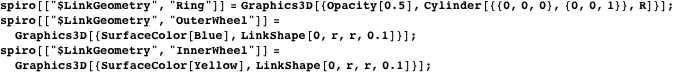The spirograph mechanism is fully defined, having one degree of freedom, which is represented in thedriving variable. The variablesout andin became explicitly derived parameters as a result of the ReplaceDrivingVariables function. The spirograph mechanism describes a family of similar mechanisms differing only in the substitution value of the geometric parameters. To select one mechanism from the family, set a new numerical value to the parameter that will be substituted in the numerical calculations.

Set the radii of Ring and the Wheels with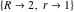.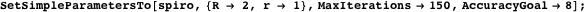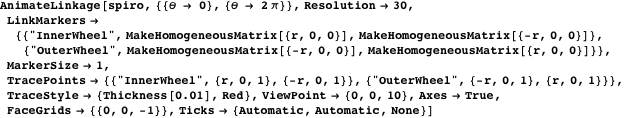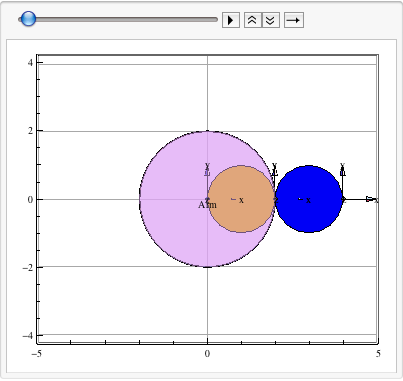### Inverse Kinematics with Replacements

The solution of the IKP is one of the most challenging problems in manipulator design. The problem is formulated as follows: given the desired position and orientation of the tool relative to the reference coordinate frame, calculate the set of joint angles that moves the tool into this posture. Numerous solution techniques have been developed that range from numerical solutions to closed form solutions. For a summary of the existing techniques, consult any standard textbook on robotics [4, 5]. In this section, a shortcut solution will be presented that enables the designer to quickly solve the inverse kinematic equation. This solution is based on simple replacements of the driving variables and utilizes the same ReplaceDrivingVariables function that was employed in the previous section.

#### Serial Manipulator

The 6R manipulator shown in Figure 4 is generated with the presented Denavit and Hartenberg (D-H) parameters. The manipulator is an open chain mechanism; therefore, the embedded method does not generate constraint equations. The manipulator has six degrees of freedom that are represented by the driving variables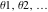. In the inverse problem, we would like to “drive” the mechanism by commanding the tool with regard to the Cartesian reference frame. This leads to the idea that if the position and orientation of the tool are parameterized, these parameters could become the new driving values of the mechanism and the old ones should be constrained with equations containing the new driving variables. This way of setting the substitution value for the new driving variables and then solving the constraint equations of the IKP would result in the substitutional values of the joint variables, which in turn are substituted into the homogeneous transformation, and the new posture of the mechanism is calculated.

To follow this process, the mechanism definition is not presented here; the predefined mechanism will be loaded, and only the replacement procedure is discussed in detail.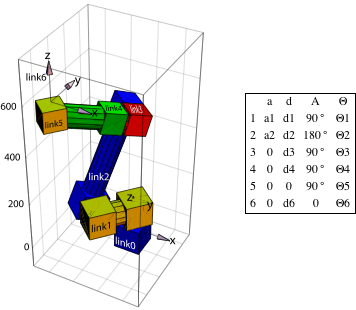Figure 4. 6R manipulator.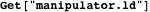In order to solve the IKP, the equation should first be defined. In this example, the local reference frame of link6 (see Figure 4) is considered as the tool frame. This selection is arbitrary, but the method would work for any other tool frame. The new driving variables are the position vector of the origin and the Euler angles of the orientation of this frame, denoted by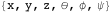. To generate the constraint equation, the homogeneous transformation matrix of link6 is calculated with respect to the world reference frame Ground.

Retrieve the transformation matrix of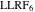.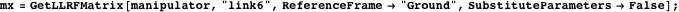Extract the position vector of the origin from mx and make it equal to the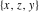vector.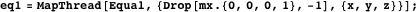The orientation of a frame is coded in the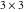rotation matrix part of the homogeneous matrix. The rotation matrix is an orthonormalmatrix that can be represented with three independent parameters. In the literature there are many rotation matrix representations such as Euler angles, Euler parameters, Rodriguez parameters, and roll-yaw-pitch. These are equivalent representations; therefore, we could pick any of them to use as driving variables in the IKP. From a technical point of view, the Rodriguez parameters are the easiest to calculate from a given rotation matrix. Therefore, the generation of the constraint equation for the orientation of the tool is done in three steps.

1. Select the rotation matrix representation (e.g., Euler angles) and define the rotation matrix using the parameters of the selected representation. This matrix is called rotmx1.
2. Extract the rotation matrix from the tool matrix. This matrix is called rotmx2.
3. Extract the Rodriguez parameters from rotmx1 and rotmx2 and make them equal.

Define the ExtractRodriguezParameters function.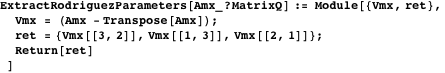Define the rotation matrix with the new driving variables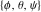based on the Euler angles parametrization.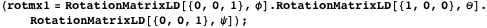Extract the rotation matrix from thehomogeneous matrix.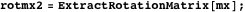Create the constraint equation for the orientation of the tool.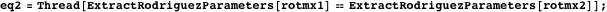Replace the old driving variables of the manipulator with the parameter, specifying the position and orientation of the tool.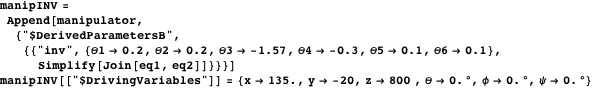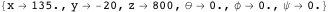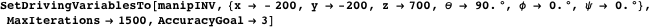Generate a substitution list for the manipulator’s parameter, as the IKP parameters are interpolated along a path.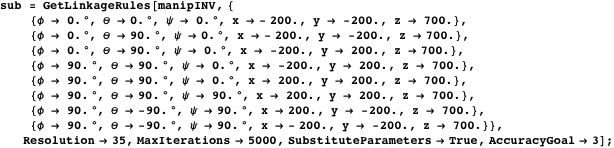Plug in the calculated joint variables of the original (direct manipulator) to visually check the result of the calculation (to minimize the size of the notebook, only every tenth interpolation point is used in the animation).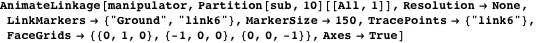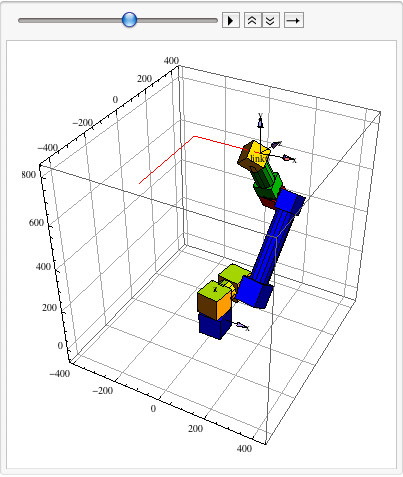Plot the axis interpolation as the tool follows the prescribed path.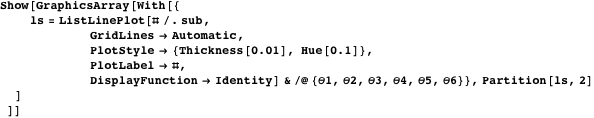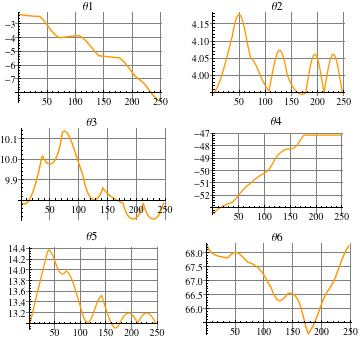#### Parallel Manipulator

Unlike serial manipulators, parallel manipulators contain loops in their kinematic graph; therefore, during the modeling phase, constraint equations are created. The process presented for the serial manipulator that quickly calculated the IKP could be applied to this case in exactly the same way. The manipulator is patented by NASA (U.S. Patent No. 5,816,105). The mechanism has three degrees of freedom with three loops in the kinematic graph (see Figure 5).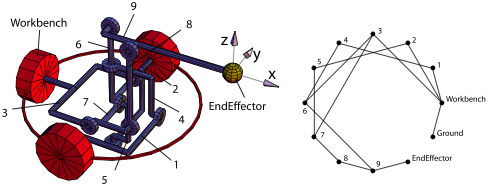Figure 5. NASA Parallel manipulator.

The mechanism definition is not presented here; the predefined mechanism will be loaded and only the replacement procedure is discussed in detail.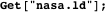Here is the list of defined constraint equations.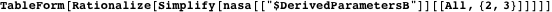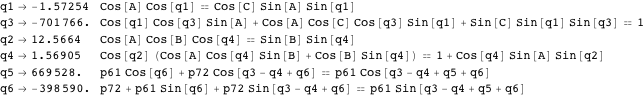Here are the simple geometric parameters of the mechanism.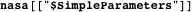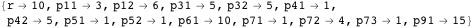The IKP in this case takes only the position of the end-effector as input because the mechanism has only three degrees of freedom and cannot specify the position and orientation together. The output of the IKP is the values of the independent joint variables, which is the rotational joint defined on motor 3 of the Workbench link in Figure 5. To define the IKP, the homogeneous transformation matrix of the tool marker should be calculated.

Get the homogeneous transformation matrix of EndEffector.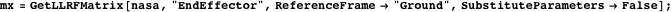Unlike the serial manipulator, the parameters presented in the transformation matrix are not all independent because some of them are already constrained by the loop-closing constraint equations as listed in the \$DerivedParametersB record. Fortunately, this does not cause a problem because the ReplaceDrivingVariables function appends the constraint equations of the IKP to the \$DerivedParametersB record. In the case of changing independent parameters (stored in the \$DrivingVariables and \$SimpleParameters records), the solver lumps together all constraint equations and solves them. Thus all constraints imposed either by loop-closing or IKP are satisfied.

Calculate the constraint equation of the IKP.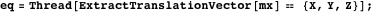Replace the driving variables with the parameters of the IKP (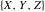).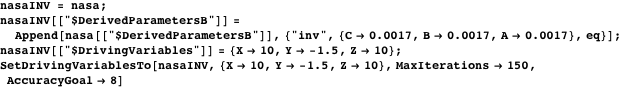Generate a substitution list for the mechanism’s parameters as the IKP parameters are interpolated along a path.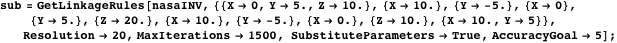Plug in the calculated joint variables of the original (direct mechanism) to visually check the result of the calculation (to minimize the size of the notebook, only every tenth interpolation point is used in the animation).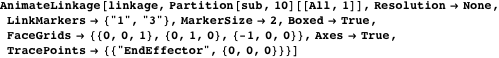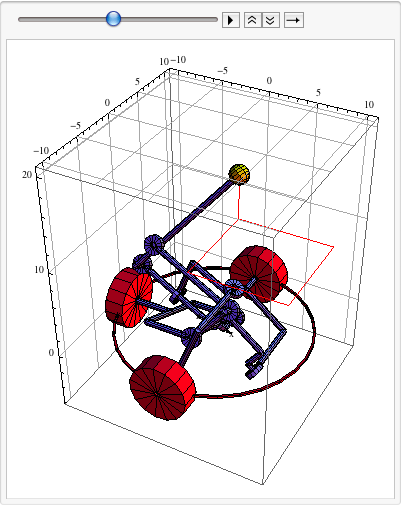Plot the axis interpolation as EndEffector follows the prescribed path.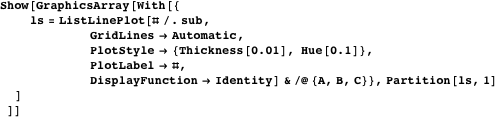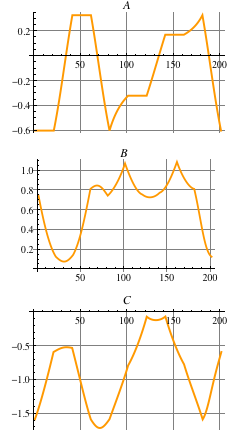### Conclusion

The embedded method works with a minimal set of constraint equations that are automatically generated. It represents the mechanism as a graph that enables measuring the relative transformation of two arbitrary points or frames of the mechanism. These two features make this method very attractive for use in mechanism prototyping because any design equation can be easily generated in a parameterized form that could be further processed by Mathematica to arrive at the optimized substitution values of the parameters. Once the design is defined and optimized, the mathematical model of the resulting mechanism is defined as a set of parameterized transformations and constraints and a list of substitution values of the parameters.

### Acknowledgment

This research was partially funded by the VRL-KCIP (FP6-507487-2) European project. This support is gratefully acknowledged.

### References

  E. J. Haug, Computer Aided Kinematics and Dynamics of Mechanical Systems (Allyn and Bacon Series in Engineering), Englewood Cliffs, NJ: Prentice-Hall College Division, 1989.  R. A. Wehage and E. J. Haug, “Generalized Coordinate Partitioning for Dimension Reduction in Analysis of Constrained Dynamic Systems,” ASME Journal of Mechanical Design, 104(1), 1982 pp. 247-255.  B. Szoke, I. Lipka, G. Petrich, and G. Szoke, “Exchange of Views in Letters Concerning a Construction Having the Principle of Parabola-Drawing Device,” KGM Bulletin of Machine Tools Works, 17(2), 1977 pp. 21-46.  J. J. Craig, Introduction to Robotics: Mechanics and Control, 2nd ed., Reading, MA: Addison-Wesley Publishing Company, 1989.  R. P. Paul, Robot Manipulators: Mathematics, Programming, and Control (Artificial Intelligence), Cambridge, MA: MIT Press, 1981. G. Erdos, “Substitutions and Replacements in Mechanism Prototyping,” The Mathematica Journal, 2011. dx.doi.org/doi:10.3888/tmj.11.2-7.